Python 中的物件導向程式設計 (OOP)：Python 中的這種範例圍繞著建立可重複使用程式碼。它涉及使用類別作為物件的藍圖。這些物件可以具有定義其行為和交互的屬性（資料）和方法（函數）。

Python 的 Magic/Dunder 方法：Python 中的 Magic 或 Dunder（雙底線）方法是名稱以雙底線開頭和結尾的特殊方法（例如，`__init__``__str__``__repr__`）。

https://dev.to/swirl/python-mastery-pythons-object-oriented-programming-overview-and-fundamentals-22m1

## 開源 Python 專案：Swirl• Python

• 人工智慧

• 在任何產品中整合大型語言模型

• 了解如何開發搜尋平台。

https://github.com/swirlai/swirl-search

https://github.com/swirlai/swirl-search

### 基本鍊錶

Next：指向下一個資料節點或儲存下一個資料節點的位址。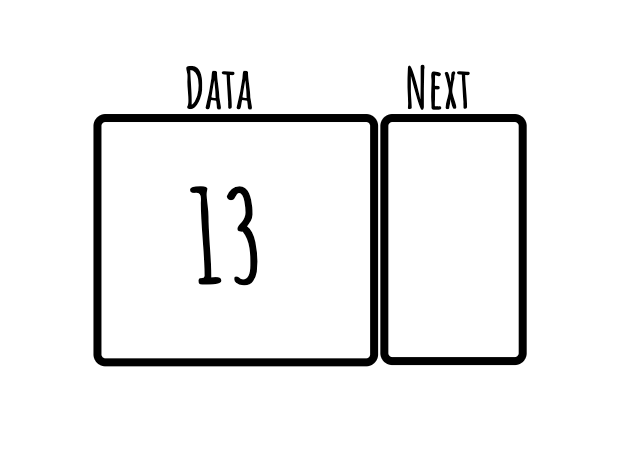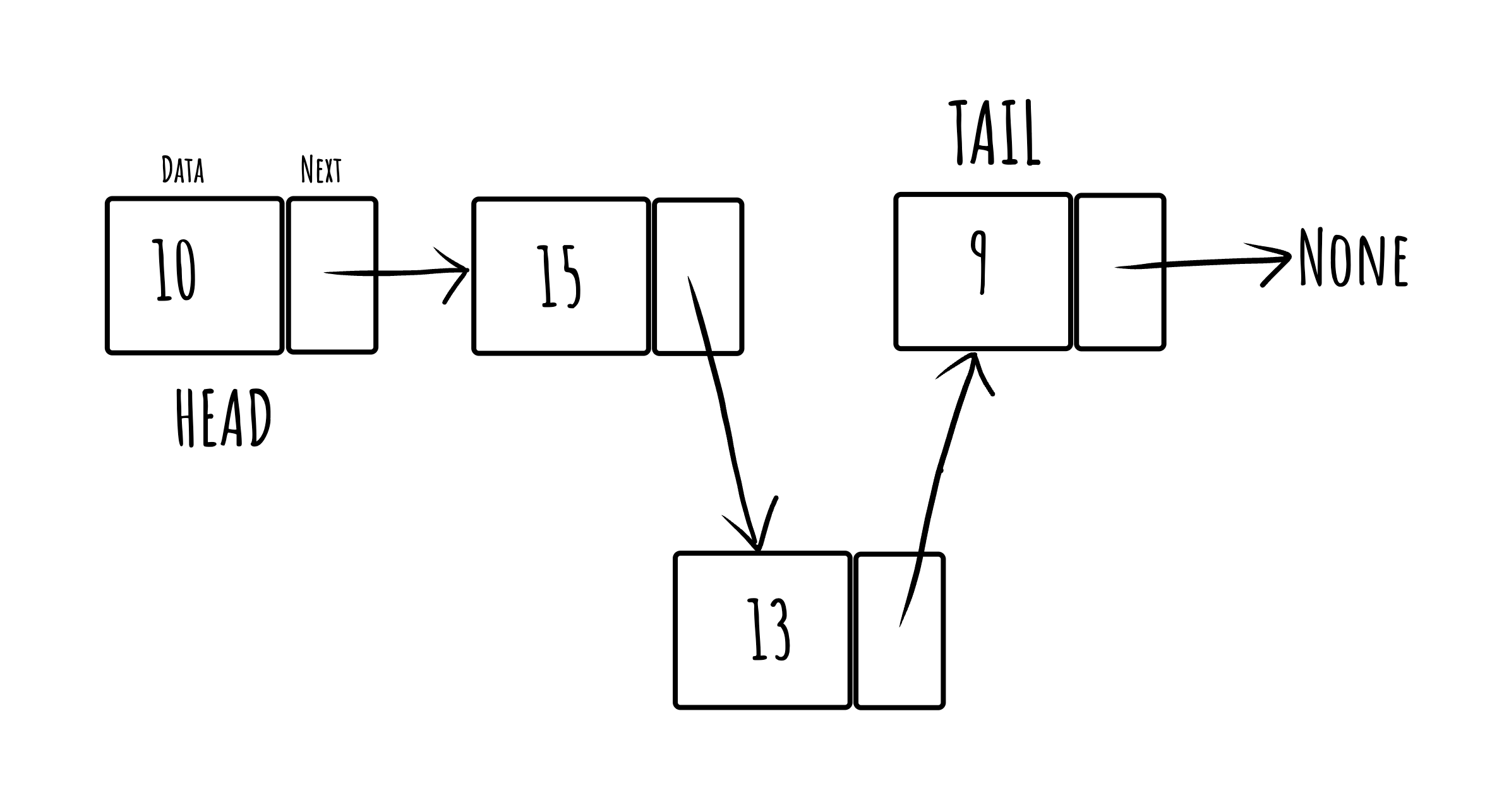### 鍊錶的好處和操作的時間複雜度

1. 動態大小：與陣列不同，鍊錶可以動態增長或縮小大小，這對於記憶體使用來說是高效的。

2. 易於插入/刪除：插入或刪除節點相對簡單，因為它通常只涉及更改一些引用，而不需要像陣列中那樣移動元素。

3. 靈活性：它們可以實現其他資料結構，如堆疊、佇列和圖鄰接表。

## 在 Python 中實作鍊錶。

``````class Node:
def __init__(self, data):
self.data = data
self.next = None

def __repr__(self):
return f"Node({self.data})"``````

``````class LinkedList:
def __init__(self):

def append(self, data):
new_node = Node(data)
return
while last_node.next:
last_node = last_node.next
last_node.next = new_node

def __repr__(self):
nodes = []
while current:
nodes.append(repr(current))
current = current.next
return "->".join(nodes)``````

1. 追加：在鍊錶末端追加一個節點。

2. `__repr__` ：此方法遍歷鍊錶並以Pythonic方式列印它。

3. 這也可以使用稱為 traverse 的方法來完成。

_這是呼叫「repr」方法的「print(llist)」的輸出：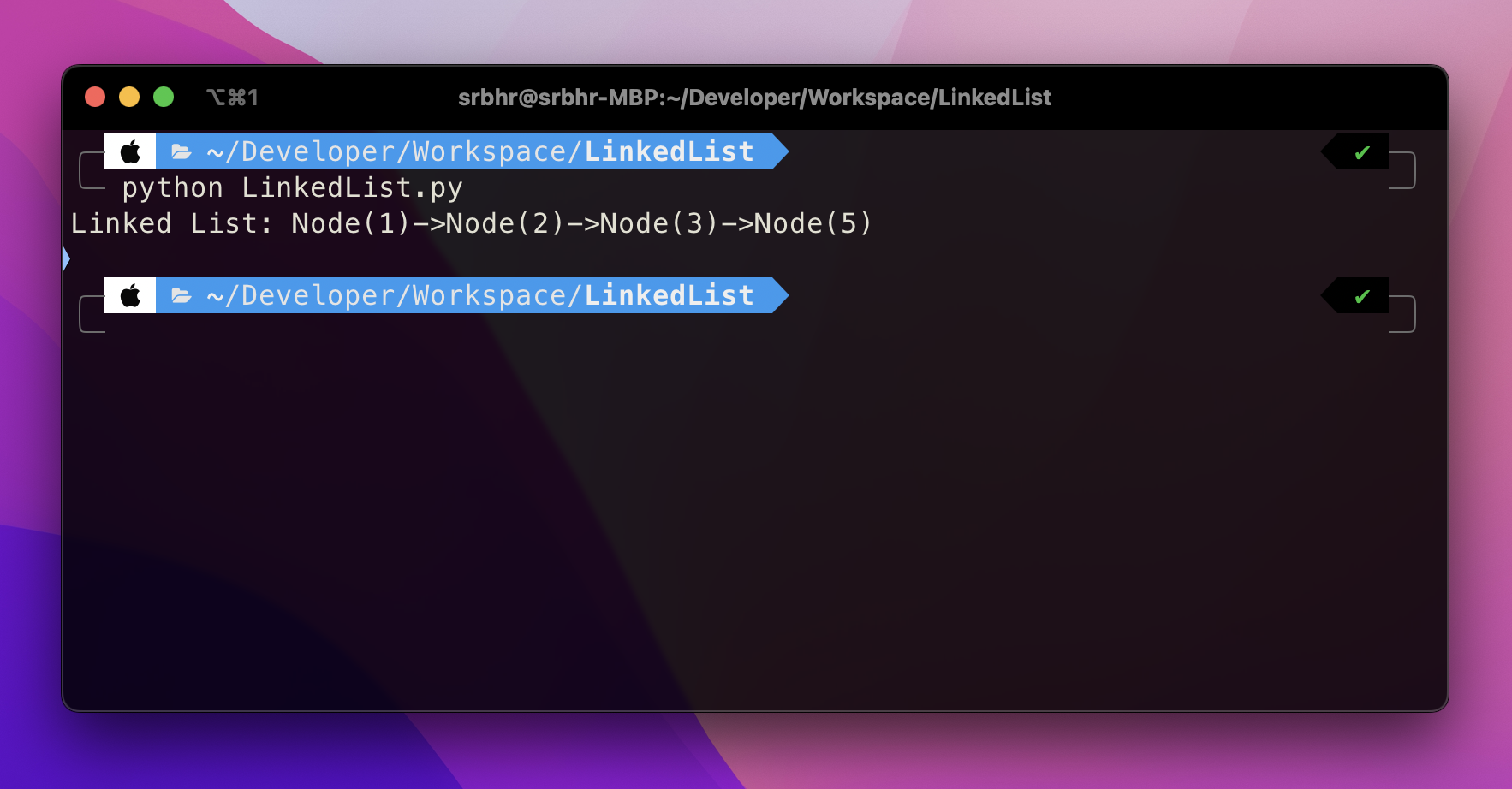### 遍歷鍊錶。

``````def traverse(linked_list):
while current:
print(current.data)
current = current.next

llist.append(1)
llist.append(2)
llist.append(3)

traverse(llist)
``````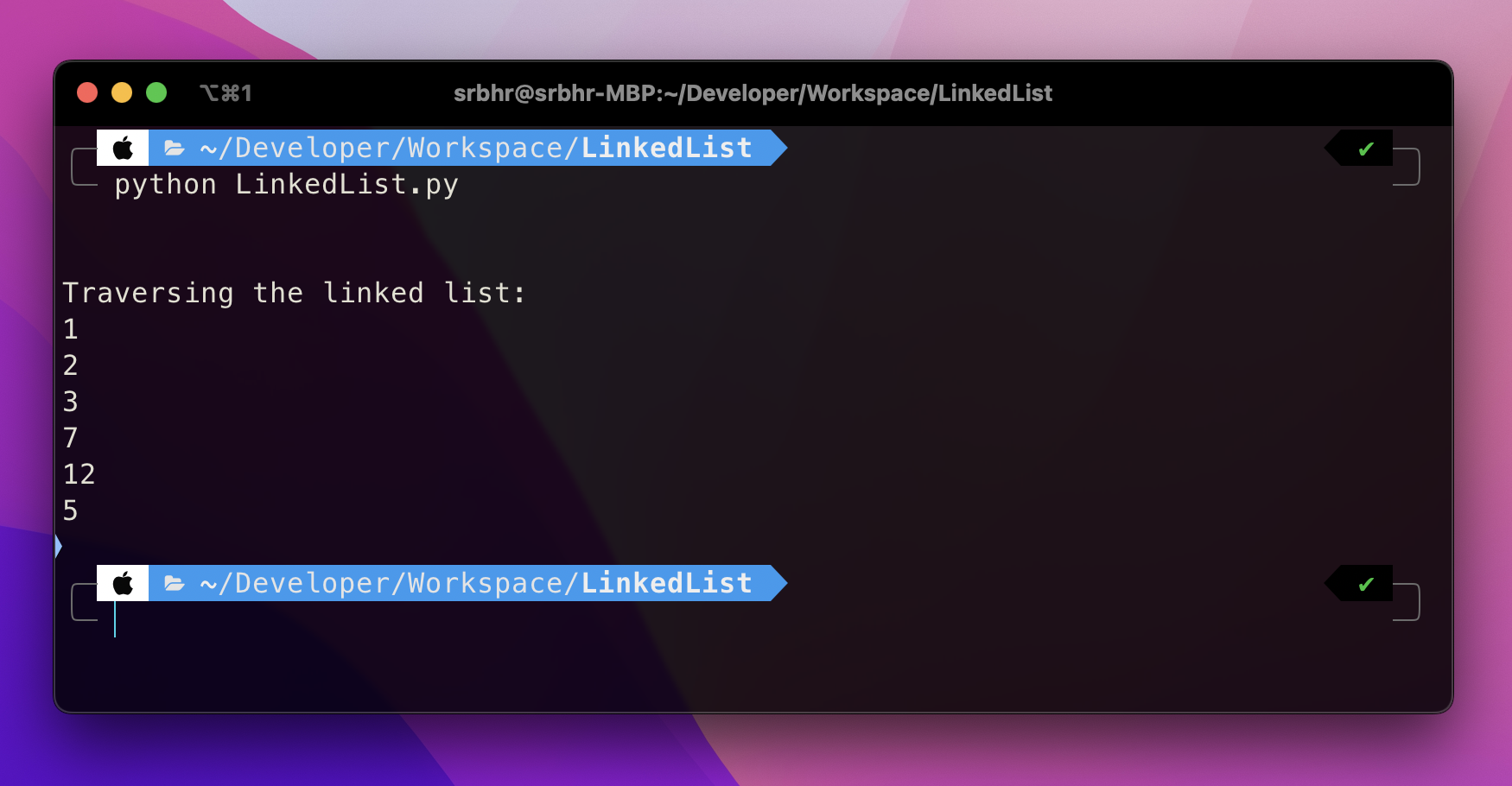## 反轉鍊錶

``````def reverse_linked_list(head):
previous = None
while current:
next_node = current.next
current.next = previous
previous = current
current = next_node
return previous

llist.append(1)
llist.append(2)
llist.append(3)
print("Original List:", llist)

print("Reversed List:", llist)
``````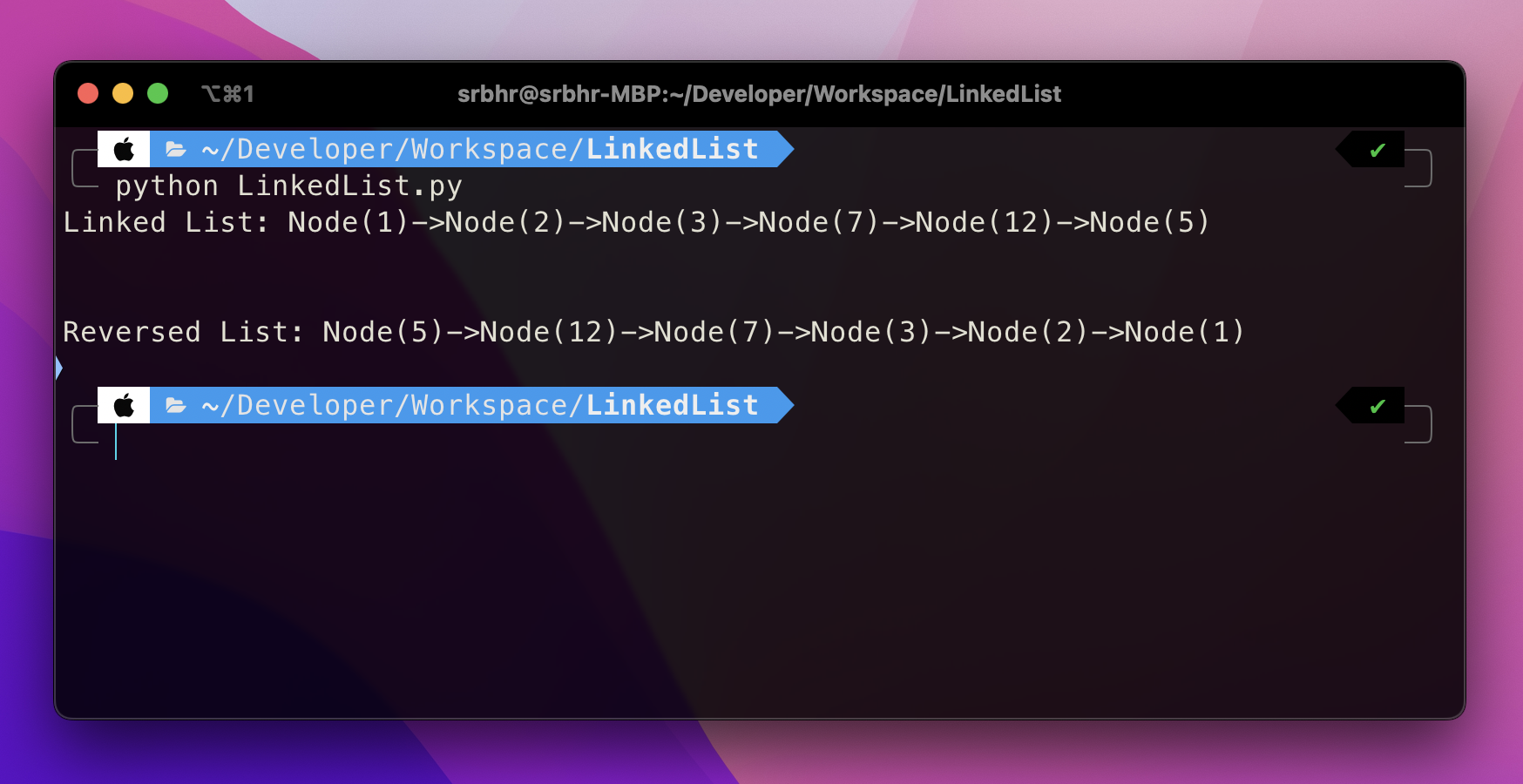## 在連結清單中插入值

``````class LinkedList:

def insert_after_value(self, data_after, data_to_insert):
return

while current:
if current.data == data_after:
new_node = Node(data_to_insert)
new_node.next = current.next
current.next = new_node
return
current = current.next

self.append(data_to_insert)
``````

## 刪除鍊錶中的節點

``````class LinkedList:

def delete_node(self, data):

if current is None or current.data == data:
self.head = current.next if current else None
return

while current.next:
if current.next.data == data:
current.next = current.next.next
return
current = current.next``````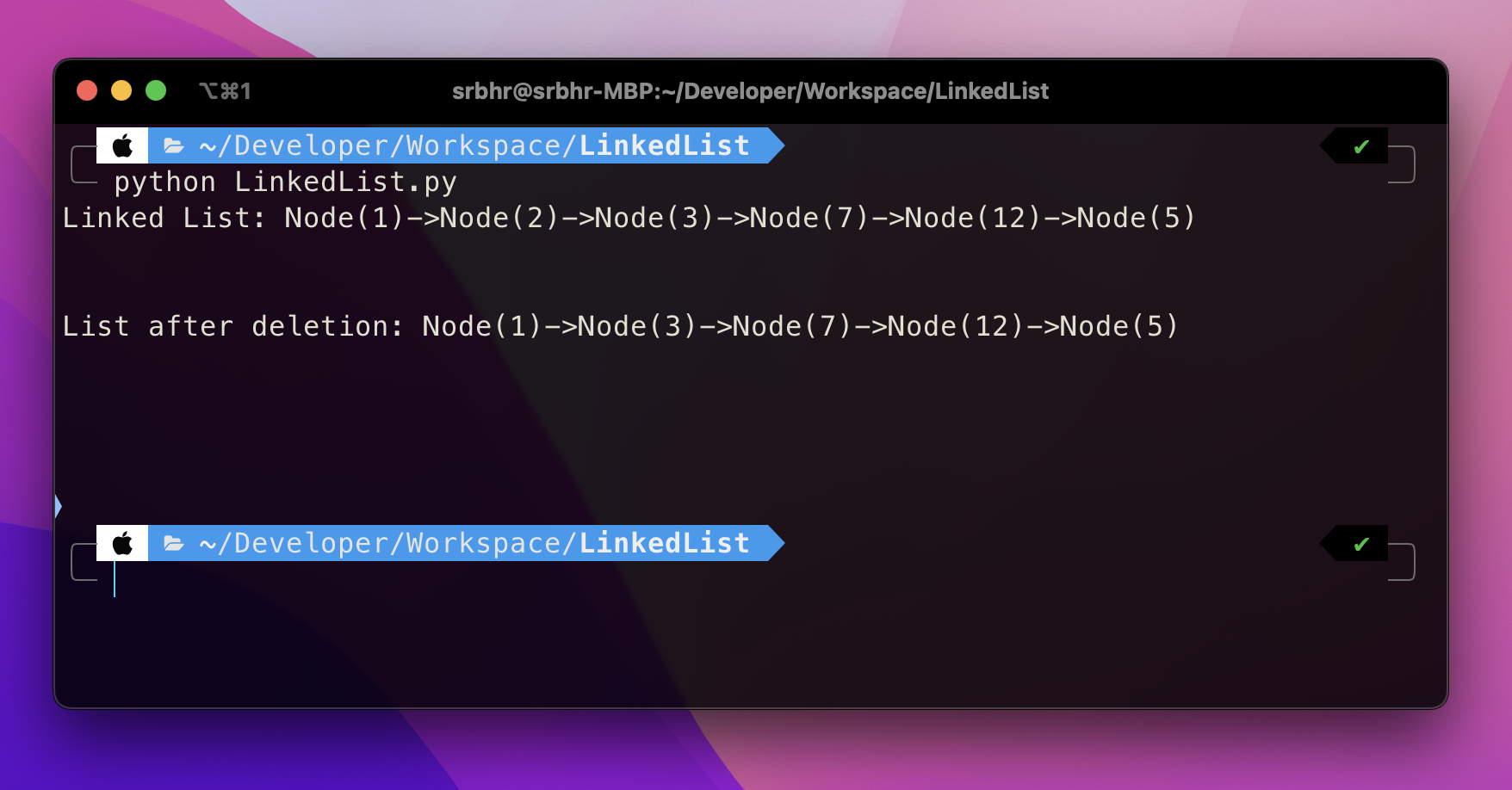## 為 [Swirl] 做出貢獻(https://github.com/swirlai/swirl-search)Swirl 是一個開源 Python 專案。為 Swirl 做出貢獻可以幫助您獲得生產級的 Python 知識並提高您的技能。

https://github.com/swirlai/swirl-search

https://github.com/swirlai/swirl-search